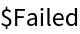#Function Repository Resource:

# ListD

Find the numerical derivative of a list of values or pairs of values

Contributed by: Jon McLoone  |  Jon McLoone
 ResourceFunction["ListD"][data] finds the derivative of a list of data. ResourceFunction["ListD"][data,type] finds the derivative of a list of data using the method type.

## Details and Options

Available choices for method are "Forward", "Backward", "Center" or "Fourier".
"Forward", "Backward" and "Center" all use linear fitting on a window of points with the given relative position to the point being considered.
With "WindowSize"1 the values computed by "Forward", "Backward" and "Center" are simply the quotient of differences in the values.
The "Fourier" method uses an FFT based method but is only supported for regularly spaced points.
One dimensional data is assumed to be regularly spaced with unit spacing.
 "WindowSize" 1 the number of extra points to use to find a linear fit

## Examples

### Basic Examples (2)

Find the derivative of data sampled from a function:

 In:=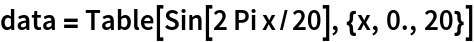Out=In:=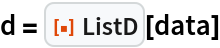Out=In:=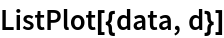Out=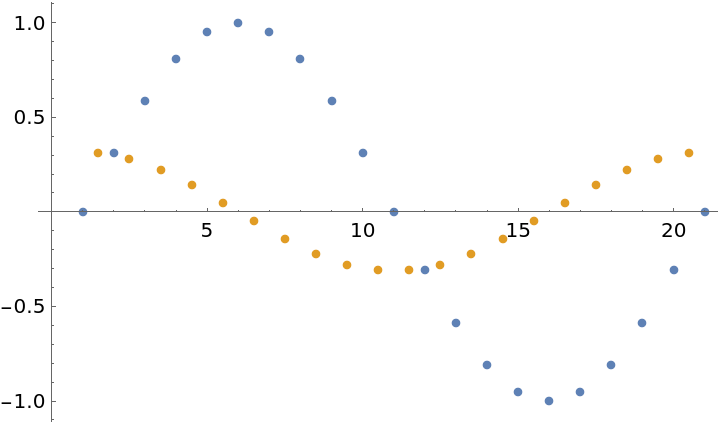Find the derivative of {x,y} data sampled from a function:

 In:=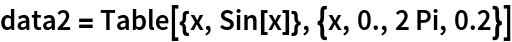Out=In:=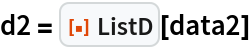Out=In:=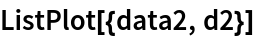Out=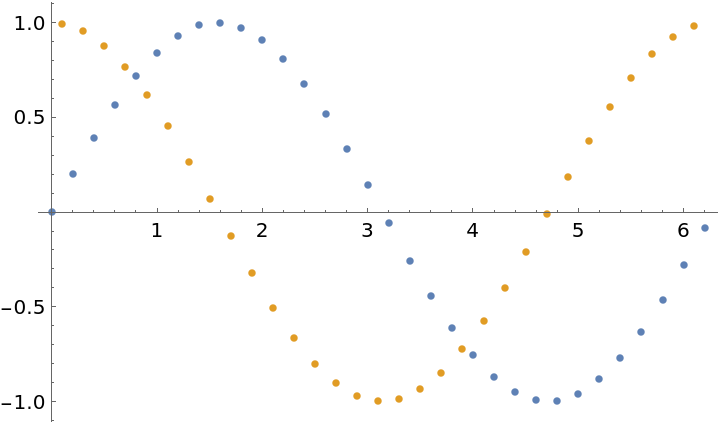### Scope (4)

The default method, "Center" generates new points between each of the original x-values using values from both sides to approximate the derivative:

 In:=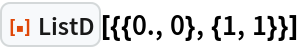Out=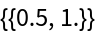The method, "Forward" generates new points at each of the original x-values using the next values to determine the derivative:

 In:=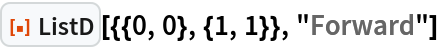Out=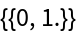The method, "Backward" generates new points at each of the original x-values using the previous values to determine the derivative:

 In:=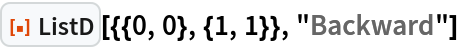Out=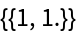The method, "Fourier" is appropriate for larger sets of oscillatory data:

 In:=In:=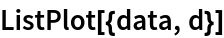Out=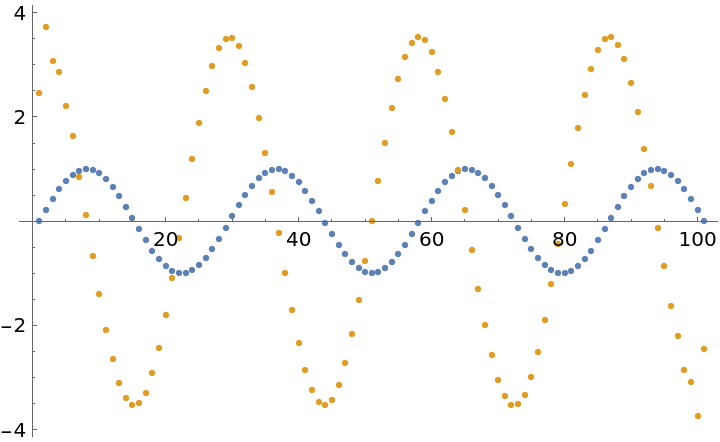### Options (3)

A small amount of noise in data can create significant errors in ListD:

 In:=Out=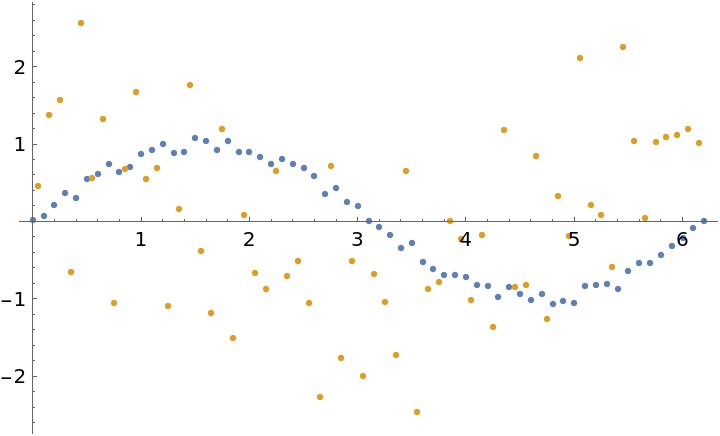By increasing the "WindowSize" the derivative is established by from the best local fit to the points:

 In:=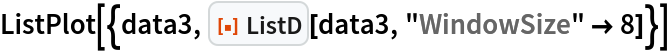Out=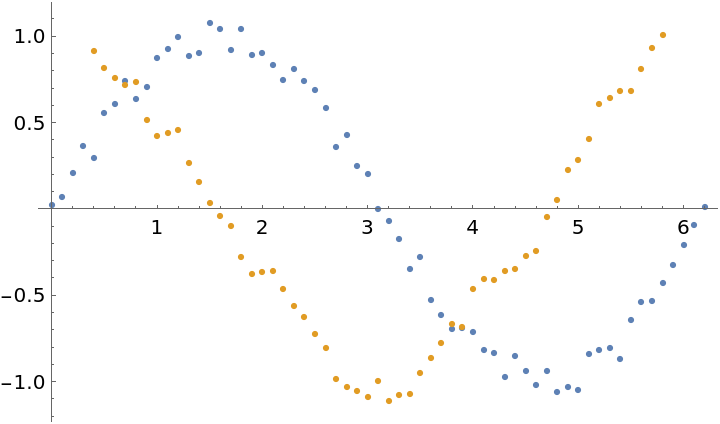Smoothing the data first to remove noise has a similar effect but often produces less smooth results for the same amount of smoothing:

 In:=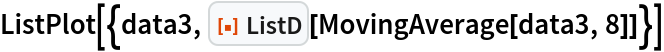Out=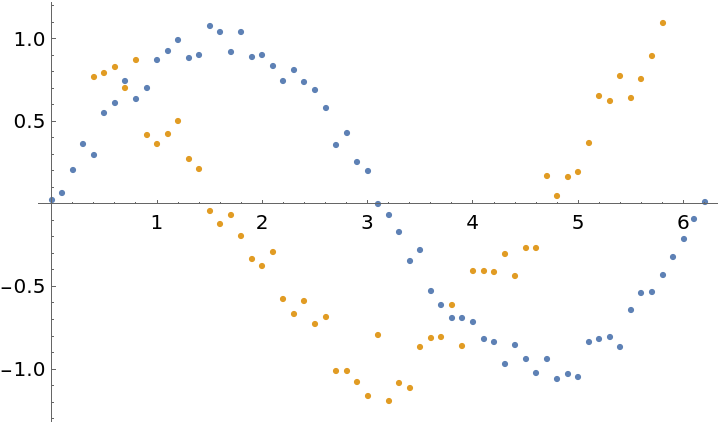### Possible Issues (1)

The "Fourier" method is only supported for regularly sampled data:

 In:=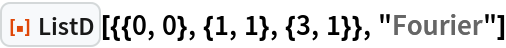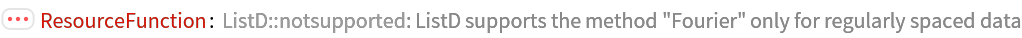Out=